# MP Board Class 10th Maths Solutions Chapter 3 Pair of Linear Equations in Two Variables Ex 3.4

In this article, we will share MP Board Class 10th Maths Book Solutions Pair of Linear Equations in Two Variables Ex 3.4 Pdf, These solutions are solved subject experts from the latest edition books.

## MP Board Class 10th Maths Solutions Chapter 3 Pair of Linear Equations in Two Variables Ex 3.4

Question 1.
Solve the following pair of linear equations by the elimination method and the substitution method:
(i) x + y = 5 and 2x – 3y = 4
(ii) 3x + 4y = 10 and 2x – 2y = 2
(iii) 3x – 5y – 4 = 0 and 9x = 2y + 7
(iv) $$\frac{x}{2}+\frac{2 y}{3}$$ = -1 and $$x-\frac{y}{3}$$ = 3
Solution:
(i) Elimination method :
x + y = 5 … (1)
2x – 3y = 4 …. (2)
Multiplying (1) by 3, we get
3x + 3y = 15 …. (3)
Adding (2) and (3) , we get
2x – 3y + 3x + 3y = 19
⇒ 5x = 19 ⇒ x = $$\frac{19}{5}$$
Now, putting x = $$\frac{19}{5}$$ in (1) , we get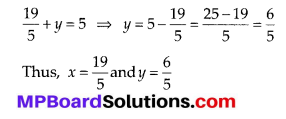Substitution Method :
x + y = 5 ⇒ y = 5 – x … (1)
2x – 3y = 4 … (2)
Put y = 5 – x in (2), we get
2x – 3(5 – x)= 4 ⇒ 2x – 15 + 3x = 4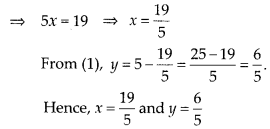(ii) Elimination method :
3x + 4y = 10 … (1)
2x – 2y = 2 … (2)
Multiplying equation (2) by 2, we have
⇒ 4x – 4y = 4 … (3)
Adding (1)and (3) , we get
3x + 4y + 4x – 4y = 10 + 4
⇒ 7x = 14 ⇒x = $$\frac{14}{7}$$ = 2
Putting x = 2 in (1) , we get,
3(2)+ 4y = 10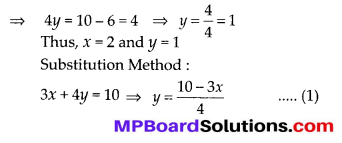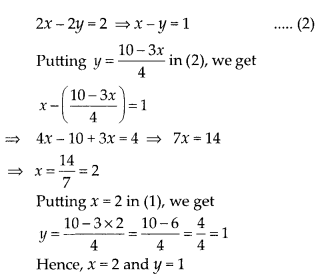(iii) Elimination method :
3x – 5y – 4 = 0 … (1)
9x = 2y + 7 ⇒ 9x – 2y – 7 = 0 …. (2)
Multiplying equation (1) by (3) , we get
⇒ 9x – 15y – 12 = 0(3)
Subtracting (2)from (3),
⇒ 9x – 15y – 12 – 9x + 2y + 7 = 0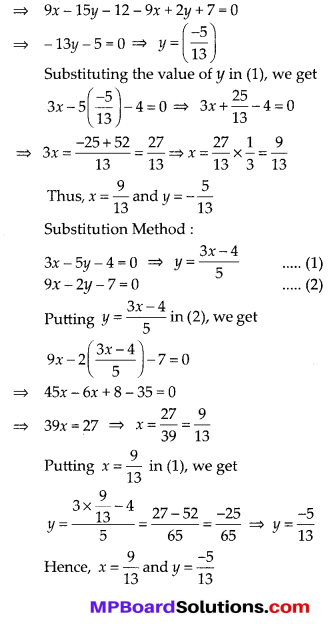(iv) Elimination method :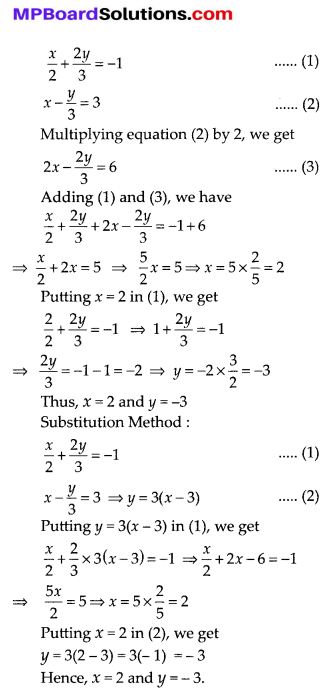Question 2.
Form the pair of linear equations in the following problems and find their solutions (if they exist) by the elimination method:
(i) If we add 1 to the numerator and subtract 1 from the denominator, a fraction reduces 1
to 1. It becomes $$\frac{1}{2}$$ if we only add 1 to the denominator. What is the fraction?
(ii) Five years ago, Nuri was thrice as old as Sonu. Ten years later, Nuri will be twice as old as Sonu. How old are Nuri and Sonu?
(iii) The sum of the digits of a two-digit number is 9. Also, nine times this number is twice the number obtained by reversing the order of the digits. Find the number.
(iv) Meena went to a bank to withdraw ₹ 2000. She asked the cashier to give her ₹ 50 and ₹ 100 notes only. Meena got 25 notes in all. Find how many notes of ₹ 50 and ₹ 100 she received.
(v) A lending library has a fixed charge for the first three days and an additional charge for each day thereafter. Saritha paid ₹ 27 for a book kept for seven days, while Susy paid ₹ 21 for the book she kept for five days. Find the fixed charge and the charge for each extra day.
Solution:
(i)Let the numerator = x
and the denominator = y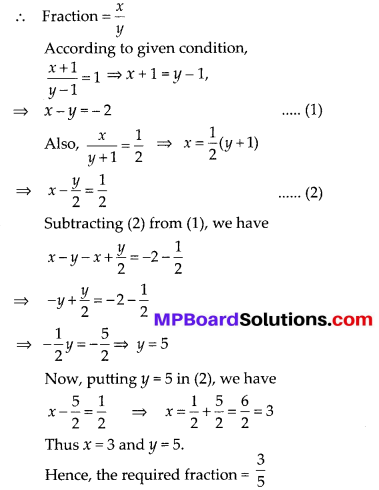(ii) Let, present age of Nuri = x years
and the present age of Sonu = y years
Age of Nuri = (x – 5) years
Age of Sonu = (y – 5) years
According to question:
Age of Nuri = 3[Age of sonu]
⇒ x – 5 = 3[y – 5] ⇒ x – 5 = 3y – 15
⇒ x – 3y + 10 = 0 … (1)
10 years later :
Age of Nuri = (x + 10) years,
Age of Sonu = (y + 10) years,
According to question :
Age of Nuri = 2[Age of Sonu]
⇒ x + 10 = 2(y + 10) ⇒ x + 10 = 2y + 20
⇒ x – 2y -10 = 0 …. (2)
Subtracting (1) from (2),
x – 2y – 10 – x + 3y – 10 = 0 ⇒ y – 20 = 0 ⇒ y = 20
Putting y = 20 in (1), we get
x – 3(20) + 10 = 0 ⇒ x – 50 = 0 ⇒ x = 50
Thus, x = 50 and y = 20
∴ Age of Nuri = 50 years
and age of Sonu = 20 years(iii) Let the digit at unit’s place = x
and the digit at ten’s place = y
∴ The number = 10y + x
The number obtained by reversing the digits = 10x + y
According to question,
9[The number] = 2 [Number obtained by reversing the digits]
9[10y+ x] = 2[10x + y]
90y + 9x = 20x + 2y
x – 8y = 0 …. (1)
Also sum of the digits = 9
x + y = 9 …. (2)
Subtracting (1) from (2), we have
x + y – x + 8y = 9
⇒ 9y = 9 ⇒ y = 1
putting y = 1 in (2), we get
x + 1 = 9 ⇒ x = 8
Thus, x = 8 and y = 1
∴ The required number = 10y + x = (10 × 1) + 8 = 10 + 8 = 18(iv) Let the number of 50 rupees notes = x
and the number of 100 rupees notes = y
According to the condition,
Total number of notes = 25
∴ x + y = 25 …. (1)
Also, the value of all the notes = ₹ 2000
∴ 50x + 100y = 2000 ⇒ x + 2y = 40 …. (2)
Subtracting equation (1) from (2), we get
x + 2y – x – y = 40 – 25
⇒ y = 15
Putting y = 15 in (1),
x + 15 = 25
⇒ x = 25 – 15 = 10
Thus, x = 10 and y = 15
∴ Number of 50 rupees notes = 10
and number of 100 rupees notes = 15

(v) Let the fixed charge (for the three days) = ₹ x
and the additional charge for each extra day = ₹ y
According to question,
Charge for 7 days = ₹ 27
⇒ x + 4y = 27
[∵ Extra days = 7 – 3 = 4]
Also, charge of 5 days = ₹ 21
⇒ x + 2y = 21
[∵ Extra days = 5 – 3 = 2]
Subtracting (2) from (1), we get
x + 4y – x – 2y = 27 – 21
$$\Rightarrow \quad 2 y=6 \Rightarrow y=\frac{6}{2}=3$$
Putting y = 3 in (2), we have
x + 2(3) = 21
⇒ x = 21 – 6 = 15
So, x = 15 and y = 3
∴ Fixed charge = ₹ 15
and additional charge per day = ₹ 3